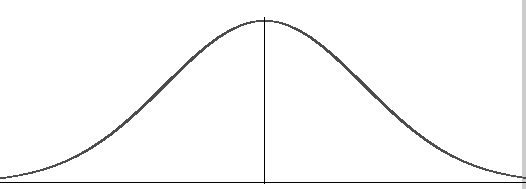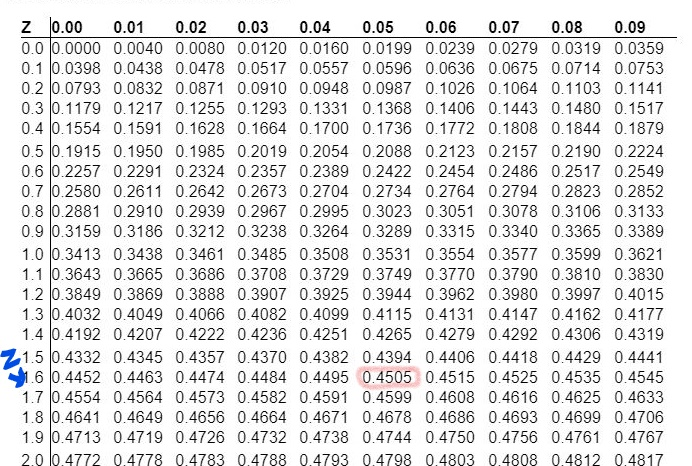prpri Calculation of Value At Risk (VAR) Calculation of Value At Risk (VAR)

# Calculation of Value At Risk (VAR)

WHAT IS VAR?

Value at Risk is basically a statistical tool to measure the expected loss at a particular time period from particular Stock or Whole Portfolio with given Confidence Level (Probability Level).

Say for Example, Mr. A wants to invest 2,00,000 in Stock of ABC Co. for 1 day S.D. of Stock is Say 5%. Mr. A wants to know the expected loss he can incur with 99% confidence level.

REQUIREMENTS FOR CALCULATION OF VAR?

1. Standard Deviation of Stock (Standard Deviation means a movement of Stock on-an-average at particular time frame)

2. Confidence level (We have to decide, at what confidence level we want to calculate expected loss)

3. Time Period (Time Period for which we want to invest in particular Stock.

4. Amount of Investment

5. Normal Distribution Table (To calculate Z Value)

FORMULA FOR VAR

VAR= S.D IN VALUE * Z VALUE

Where

S.D. in Value = Investment Amount*S.D of Stock

Z Value= No of S.D. from Mean (This we have see from Normal Distribution Table)

Continuing our Example,

Lets Calculate Expected Loss for Mr. A

VAR= S.D IN VALUE * Z VALUE

S.D. in Value= 2,00,000*5%= 10,000

VAR= 10000*Z Value______________________________________

———–45%————  ————50%—————–

We have to see now Z Value for 45% in Normal Distribution Table,We Got Z Value for 45% is 1.6

Note= For 100% Accuracy interpolation between 0.4505 and 0.4495 can be done.

VAR= 10,000 (S.D. in Value) * 1.6 (Z Value)

=16000.

Interpretation:

Mr. A can invest Rs. 2,00,000 for 1 Day with expected loss of 16,000 with 95% Confidence Level.

In other words, It is 95% probability that loss of Mr. A will not be exceeding 16,000 and 5% probability of incurring loss of more than 16,000 respectively.

#### Author Bio

Qualification: Student - CA/CS/CMA
Company: N/A
Location: Maharashtra, IN
Member Since: 13 Dec 2020 | Total Posts: 7
I am CA final Student, currently doing my Articleship from Mid sized firm. My CA final Attempt is due in May 2021. I am Open to learning new things every time. I always relate theoretical knowledge with Practical life and it helps me alot in my CA Final Studies as well. View Full Profile

### More Under Finance

1. Arshi Haque says:

Explained in simple language..Thanks

August 2021
M T W T F S S
1
2345678
9101112131415
16171819202122
23242526272829
3031